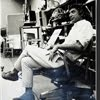## Eugene Ray SDSU 1970'sEugene Ray 1970's. photo credit: Tom Davis

## Monday, August 25, 2014

### HONORING SOME ALUMNI / nautilus & passiflora synergetic lexicon

+NAUTILUS & PASSIFLORA+
>tonight i honor thousands of fine alumni w/ a<
>small group that was assembled by our grad<
+(NAUTILUS WAS OUR SILVER+
>student jan carpenter as a special project<
>and each alum submitted an outline text<
^^^^^^^^^^^^^^^^^^^^^^^^^^^
+PASSIFLORA IS A TROPICAL+
+FLOWER I USED as SYMBOL+
^^^^^^^^^^^^^^^^^^^^^^
>alumni like dave fobes & ralph bowman<
>selected for submitting ed facebook data<
+(SEEN ON MY OWN+
^^^^^^^^^^^^^^^^^^^^^^^^^^
+A LEXICON OF VISIONARY DESIGN+
>("alternatives" environ design<
>exhibition i built at sdsu / ed)<
+TOPICS I USED IS SHOWN AS WELL+
^^^^^^^^^^^^^^^^^^^^^^^^^^^^
>the nautilus shell is nature's perfect thin<
>shell archetype for our education as to<
+(FIBONACCI SEQUENCE+
+GOLDEN MEAN DATA)+
>the excellence of biomorphic structure<
^^^^^^^^^^^^^^^^^^^^^^^^
>the tetrahedron i hold here represents another<
>perfect, although linear. structure to construct<
+OF the TETRAHEDRON)+
>buildings of great light wt. linear strength<
^^^^^^^^^^^^^^^^^^^^^^^^^
>jan carpenter, will ackell and sandi andre<
>form the initial group that jan assembled<
+(CARPENTER, ACKELL+
+ANDRE / >ED, SDSU<)+
>in a loose leaf, ribbon tied together book<
^^^^^^^^^^^^^^^^^^^^^^^^
>richard beveridge, david beck brown and<
>luis cortez were all >radiant stars< in our<
+(BEVERIDGE, BROWN+
+CORTEZ / ED, SDSU)+
>sdsu environ design diversified lexicon<
>beveridge & brown leaders in the arts<
^^^^^^^^^^^^^^^^^^^^^^^^
>david fobes, kathleen hallihan and hoby<
>hufford made my sdsu days happy w/<
+(FOBES, HALLIHAN AND+
+HUFFORD / ED, SDSU)+
>dave and kathleen especially radiant w/<
>prof dave's exquisite art, kathy's design<
>of new home w/ husband rob quigley<
^^^^^^^^^^^^^^^^^^^^^
>kevin immel, mario lara and michael lebold<
>were all stars in their individual ways w/<
+(IMMEL. LARA AND  +
+LEBOLD, (ED, SDSU)+
>kevin winning aka award, mario teaching<
>and earning a mesa college professorship<
^^^^^^^^^^^^^^^^^^^^^^^^^>cecil magpuri, yoshiki matsuhisa and<
>maureen mitton were all talented w/<
+(MAGPURI, MATSUHISA+
+& MITTON (ED, SDSU)+
>yoshi becoming a professor in japan,<
>(landscape architecture, osaka univ.)<
^^^^^^^^^^^^^^^^^^^^^^^
>kotaro nakamura, arati rangswami and<
>nozar ravenbakish were all excellent w/<
+(NAKAMURA, RANGSWAMI+
+RAVENBACH, (>ED, SDSU<)+
>kotaro presently head of sdsu art dept<
>nozar head of architecture at c.i.c.o.<
>arati is project head at smith architect<
^^^^^^^^^^^^^^^^^^^^^^^^
>lawrence uruttia were a stellar trio w/<
>brian my award winning student asst<
+& URUTTIA (ED, SDSU)+
>chikako award winning architect &<
>larry patron of my radiant concepts<
>, outstanding art exhibitions curator<
^^^^^^^^^^^^^^^^^^^^^^^
>roger utt, frank wolden & howard ziegler<
>were three great environ design students<
>roger just completing his new office and<
+(UTT,  WOLDEN   AND+
+ZIEGLER, (ED / SDSU)+
>frank & howard s.d. urban design lights<
>(frank important to horton plaza center)<
^^^^^^^^^^^^^^^^^^^^^^^^^
>in 1977 i mounted a large exhibition of our<
>student work at sdsu and sent this notice &<
+>DESIGN TOPICS< HERE)+
>invitation to our friends at the museum of<
>modern art's design division in new york<
^^^^^^^^^^^^^^^^^^^^^^^^
>yes, nature has been our best guide and<
>when one understands the magnificent<
>totality of pineal gland function to the<
+(NATURE IS OUR+
+STRONG GUIDE)+
>design concepts come bright & clear<
^^^^^^^^^^^^^^^^^^^^^^^^^
>it should be noted that expanded information<
>on our sdsu, environ design grads is found<
+/ (insert SDSU ALUMNUS)+
>on the internet & a www ref of some value<
>as i have written many sdsu alum blog refs<
^^^^^^^^^^^^^^^^^^^^^^^^^
+CONGRATS TO FRANK WOLDEN+
+FOUNDING PRINCIPAL OF THE+
+NEW S.D.>"SKYPORT STUDIO"<+
^^^^^^^^^^^^^^^^^^^^^^^^^^
>i apologize to many alumni like ed bourke<
>who have distinguished careers i would<
+(APOLOGIES TO OTHER /+
+>DESERVING< ALUMNI)+
>like to focus on and yiuman young our<
+BOB GAUGHEN etc, etc)+
>most overlooked, distinguished alum<
>(from hong kong as wun chok bong)<
^^^^^^^^^^^^^^^^^^^^^^^^^
>(many are lost to me<
>like pete peterson,<
>roger & jo marr..)<
+PEOPLE = EARTHS HARVEST+ !
^^^^^^^^^^^^^^^^^^^^^
eugene ray, mfa, architect
professor emeritus, sdsu